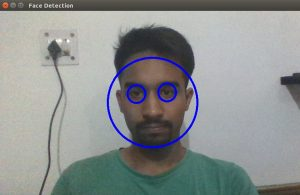# OpenCV C++ Program for Face Detection

This program uses the OpenCV library to detect faces in a live stream from webcam or in a video file stored in the local machine. This program detects faces in real time and tracks it. It uses pre-trained XML classifiers for the same. The classifiers used in this program have facial features trained in them. Different classifiers can be used to detect different objects.
Requirements for running the program:
1) OpenCV must be installed on the local machine.
2) Paths to the classifier XML files must be given before the execution of the program. These XML files can be found in the OpenCV directory “opencv/data/haarcascades”.
3) Use 0 in capture.open(0) to play webcam feed.
4) For detection in a local video provide the path to the video.(capture.open(“path_to_video”)).

Implementation:

 `// CPP program to detects face in a video ` ` `  `// Include required header files from OpenCV directory ` `#include "/usr/local/include/opencv2/objdetect.hpp" ` `#include "/usr/local/include/opencv2/highgui.hpp" ` `#include "/usr/local/include/opencv2/imgproc.hpp" ` `#include ` ` `  `using` `namespace` `std; ` `using` `namespace` `cv; ` ` `  `// Function for Face Detection ` `void` `detectAndDraw( Mat& img, CascadeClassifier& cascade,  ` `                ``CascadeClassifier& nestedCascade, ``double` `scale ); ` `string cascadeName, nestedCascadeName; ` ` `  `int` `main( ``int` `argc, ``const` `char``** argv ) ` `{ ` `    ``// VideoCapture class for playing video for which faces to be detected ` `    ``VideoCapture capture;  ` `    ``Mat frame, image; ` ` `  `    ``// PreDefined trained XML classifiers with facial features ` `    ``CascadeClassifier cascade, nestedCascade;  ` `    ``double` `scale=1; ` ` `  `    ``// Load classifiers from "opencv/data/haarcascades" directory  ` `    ``nestedCascade.load( ``"../../haarcascade_eye_tree_eyeglasses.xml"` `) ; ` ` `  `    ``// Change path before execution  ` `    ``cascade.load( ``"../../haarcascade_frontalcatface.xml"` `) ;  ` ` `  `    ``// Start Video..1) 0 for WebCam 2) "Path to Video" for a Local Video ` `    ``capture.open(0);  ` `    ``if``( capture.isOpened() ) ` `    ``{ ` `        ``// Capture frames from video and detect faces ` `        ``cout << ``"Face Detection Started...."` `<< endl; ` `        ``while``(1) ` `        ``{ ` `            ``capture >> frame; ` `            ``if``( frame.empty() ) ` `                ``break``; ` `            ``Mat frame1 = frame.clone(); ` `            ``detectAndDraw( frame1, cascade, nestedCascade, scale );  ` `            ``char` `c = (``char``)waitKey(10); ` `         `  `            ``// Press q to exit from window ` `            ``if``( c == 27 || c == ``'q'` `|| c == ``'Q'` `)  ` `                ``break``; ` `        ``} ` `    ``} ` `    ``else` `        ``cout<<``"Could not Open Camera"``; ` `    ``return` `0; ` `} ` ` `  `void` `detectAndDraw( Mat& img, CascadeClassifier& cascade, ` `                    ``CascadeClassifier& nestedCascade, ` `                    ``double` `scale) ` `{ ` `    ``vector faces, faces2; ` `    ``Mat gray, smallImg; ` ` `  `    ``cvtColor( img, gray, COLOR_BGR2GRAY ); ``// Convert to Gray Scale ` `    ``double` `fx = 1 / scale; ` ` `  `    ``// Resize the Grayscale Image  ` `    ``resize( gray, smallImg, Size(), fx, fx, INTER_LINEAR );  ` `    ``equalizeHist( smallImg, smallImg ); ` ` `  `    ``// Detect faces of different sizes using cascade classifier  ` `    ``cascade.detectMultiScale( smallImg, faces, 1.1,  ` `                            ``2, 0|CASCADE_SCALE_IMAGE, Size(30, 30) ); ` ` `  `    ``// Draw circles around the faces ` `    ``for` `( ``size_t` `i = 0; i < faces.size(); i++ ) ` `    ``{ ` `        ``Rect r = faces[i]; ` `        ``Mat smallImgROI; ` `        ``vector nestedObjects; ` `        ``Point center; ` `        ``Scalar color = Scalar(255, 0, 0); ``// Color for Drawing tool ` `        ``int` `radius; ` ` `  `        ``double` `aspect_ratio = (``double``)r.width/r.height; ` `        ``if``( 0.75 < aspect_ratio && aspect_ratio < 1.3 ) ` `        ``{ ` `            ``center.x = cvRound((r.x + r.width*0.5)*scale); ` `            ``center.y = cvRound((r.y + r.height*0.5)*scale); ` `            ``radius = cvRound((r.width + r.height)*0.25*scale); ` `            ``circle( img, center, radius, color, 3, 8, 0 ); ` `        ``} ` `        ``else` `            ``rectangle( img, cvPoint(cvRound(r.x*scale), cvRound(r.y*scale)), ` `                    ``cvPoint(cvRound((r.x + r.width-1)*scale),  ` `                    ``cvRound((r.y + r.height-1)*scale)), color, 3, 8, 0); ` `        ``if``( nestedCascade.empty() ) ` `            ``continue``; ` `        ``smallImgROI = smallImg( r ); ` `         `  `        ``// Detection of eyes int the input image ` `        ``nestedCascade.detectMultiScale( smallImgROI, nestedObjects, 1.1, 2, ` `                                        ``0|CASCADE_SCALE_IMAGE, Size(30, 30) );  ` `         `  `        ``// Draw circles around eyes ` `        ``for` `( ``size_t` `j = 0; j < nestedObjects.size(); j++ )  ` `        ``{ ` `            ``Rect nr = nestedObjects[j]; ` `            ``center.x = cvRound((r.x + nr.x + nr.width*0.5)*scale); ` `            ``center.y = cvRound((r.y + nr.y + nr.height*0.5)*scale); ` `            ``radius = cvRound((nr.width + nr.height)*0.25*scale); ` `            ``circle( img, center, radius, color, 3, 8, 0 ); ` `        ``} ` `    ``} ` ` `  `    ``// Show Processed Image with detected faces ` `    ``imshow( ``"Face Detection"``, img );  ` `} `

Output:Face Detection

Next Article: Opencv Python Program for face detection
References:

This article is contributed by Shashwat Jain. If you like GeeksforGeeks and would like to contribute, you can also write an article using contribute.geeksforgeeks.org or mail your article to contribute@geeksforgeeks.org. See your article appearing on the GeeksforGeeks main page and help other Geeks.

Attention reader! Don’t stop learning now. Get hold of all the important DSA concepts with the DSA Self Paced Course at a student-friendly price and become industry ready.

My Personal Notes arrow_drop_up
Article Tags :
Practice Tags :

9

Please write to us at contribute@geeksforgeeks.org to report any issue with the above content.